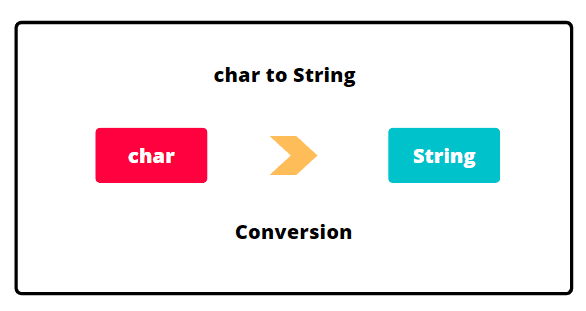# How to Convert Char to String in Java

In this tutorial, we will learn how to convert a primitive data type char to a non primitive data type string in Java easily.

Sometimes, we need to convert values from one data type to another, under certain situation.

Therefore, we will know two different ways to convert char to string in Java. Two ways for converting char to string are as follows:

• Convert using String.valueOf(char) method of String class
• Convert using Character.toString(char) method of Character class

Let’s understand both ways one by one with example programs.## Converting Char to String in Java using String.valueOf(char)

To convert primitive data type char to string in Java, we use valueOf() method of the String class. The method String.valueOf() converts char to string. It is a static utility method of the String class.

Therefore, we do not need to create an object to calling this method. We can simply call by using its class name. The general signature of valueOf() method is as below:

```public static String valueOf(char c)
```

This method accepts a char value that needs to be converted and returns the string representation of the char value.

Let’s create a simple program to convert a primitive char data type to string in Java.

Program code 1:

```// Java Program to demonstrate the conversion of char data type into string using String.valueOf().
package stringPrograms;
public class CharToString {
public static void main(String[] args)
{
char ch = 'T';
String str = String.valueOf(ch);
System.out.println("String representation of char: "+str);
System.out.println(str + 50);
System.out.println(ch + 50);
}
}
```
```Output:
String representation of char: T
T50
134
```

## Char to String Conversion using Character.toString() method

Character wrapper class in Java wraps a value of the primitive data type char in an object. An object of Character class contains a single field whose type is char.

The Character wrapper class also provides a toString() method that converts char value to String. The toString() method of Character class is a static utility method.

The general signature of this method is as below:

```public static String toString(char ch)
```

This method accepts a parameter ch that is to be converted. It returns a string object representing the specified char value.

Let’s create a simple Java program for converting primitive data type char to non primitive data type String using Character.toString() method.

Program code 2:

```// Java Program to demonstrate the conversion of char into string using Character.toString().
package stringPrograms;
public class CharToString {
public static void main(String[] args)
{
char ch = 'D';
String str = Character.toString(ch);
System.out.println("String representation of char: "+str);
System.out.println(str + 50);
System.out.println(ch + 50);
}
}
```
```Output:
String representation of char: D
D50
118
```

In this tutorial, you learned how to convert one data type char to another type String in Java. Hope that you will have understood the basic concept for converting char into string and practiced all programs.